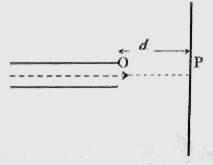## Pages

`“Life is like riding a bicycle.  To keep your balance you must keep moving.”–Albert Einstein`

## Monday, November 3, 2008

### Magnetic Force on Moving Charges- Two Questions for AP Physics B&C

"I believe in standardising automobiles, not human beings."
Albert Einstein

Questions involving magnetic fields were discussed on this site on many occasions. You can access all of them by clicking on the label ‘magnetic field’ below this post. Today we will discuss two questions involving magnetic force on moving charges:(1) A beam of positive ions of mass m, charge q and kinetic energy K proceeding along the positive x-direction emerges from the exit point O of an ion source. A plate P is placed perpendicular to the beam at a distance d from the point O (Fig.). A magnetic field is applied in the region between the point O and the plate P so that the ions are prevented from striking the plate P. Assuming that the ions are moving at non-relativistic speed, the minimum magnitude required for the magnetic field is
(a) [√(2 mK)] /qd
(b) [√(2 m2K)] /qd
(c) [√(2 K)] /qmd
(d) [√(2 mK2 )] /qd
(e) [√(2 m)] /qKd
If the speed of the ion is v, we have
K = ½ mv2 so that v =√(2K/m)
The beam will not strike the plate B if the radius of the path of the ions in the magnetic field is less than the distance d between the plate and the point O. If the magnetic field required is to be minimum, the field has to be applied perpendicular to the direction of the beam. This minimum field will make the radius R of the path equal to d. Therefore, we have
qvB = mv2/R = mv2/d so that
B = mv/qd
Substituting for v from the expression for kinetic energy we have
B = m√(2K/m)/qd = [√(2 mK)] /qd-->
(2) A sensitive electronic balance (weighing machine) carries a magnet which produces a horizontal magnetic field B tesla in the central region between its poles N and S(fig.). The reading of the balance is R newton. A horizontal beam of negatively charged particles with a constant beam current is now passed continuously through the central region between the poles of the magnet. The direction of the beam is perpendicular to the magnetic field as shown. Then the reading of the electronic balance will
(a) be R itself
(b) be less than R
(c) be greater than R
(d) go on increasing from R
(e) go on decreasing from R
The beam of negative charges proceeding rightwards, as shown in the figure, is equivalent to a beam of positive charges proceeding leftwards. The magnetic force acting on the particles is downwards (as given by Fleming’s left hand rule). The charged particles exert an equal and opposite force (reaction) on the magnet. Therefore, there is an upward force on the magnet. The electronic balance therefore shows a reading less than R.
You will find some useful questions (with solution) in this section at physicsplus